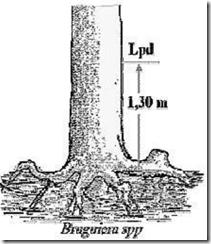Home > English > Measurement Accuracy Tree Trunk

# Measurement Accuracy Tree Trunk

When measuring the diameter or circumference is not infrequent errors. A frequent mistake is in the location of the measurement, among others:
(A) Putting a tool or when aiming (optical instrument).
(B) The reading of the scale (unit of measure).

The use of tools in the right way with adequate skills automatically constitute a correction. Similarly, when the scale reading should be done carefully. When an error occurs laying tools, may be repaired, but a mistake while reading scale can not be repaired (corrected). Therefore, the precision when measuring the diameter or circumference is preferred.

Accuracy measure the diameter or circumference (tree stand or fall) depends on several factors, among others:
(A) measuring instrument.
(B) How to measure, put a measuring instrument, skill, thoroughness desired.
(C) Time.
(D) Cost.

However, problems that often occur when the dimensional measurements of trees (diameter or circumference, height or length) is at kekurangcermatan when taking measurements. The inaccuracy affects the basal area (LBDs) and also the volume of the tree. Therefore, to improve the level of precision diameter measurement, then:
(A) The measurements were performed at least twice (except the measuring tape) remember circle trunk not a full circle.
(B) The reading of the current scale of measurement must carefully. Measurement trees (stand or fall) small diameter requires a precision that is higher than a larger diameter.

Tree wetland (swamp / brackish)
Location of measuring the diameter or circumference (LPD) on a tree trunk that grows in wetlands (swamp / brackish) is based on the type of tree. Some examples can be seen below.

• Type Bruguiera spp.
Location of measuring the diameter or circumference (LPD) on a tree trunk types Bruguiera spp. is as high as 1.30 m above the root. Measuring the diameter or circumference of the tree trunk as shown in Figure 21.Figure 21. LPD tree types Bruguiera spp.

• Type Ceriops spp.
Location of measuring the diameter or circumference (LPD) on a tree trunk types Ceriops spp. is as high as 1.30 m above the roots that borders the water. However, things that must be considered is the type of Ceriops spp. have buttressed roots that need to be reviewed in advance how much altitude the buttresses. If the height of the buttresses of less than 1.30 m, 1.30 m tall LPD do limit the roots that hit the water. Measuring the diameter or circumference of the tree trunk as shown in Figure 22.Figure 22. LPD tree types Ceriops spp.

• Type Rhizopora spp.
Location of measuring the diameter or circumference (LPD) on a tree trunk types Rhizopora spp. is as high as 0.20 m from the top end of the root portion. Measuring the diameter or circumference of the tree trunk as shown in Figure 23.Figure 23. LPD tree types Rhizopora spp."Tasikmalaya, Indonesia"